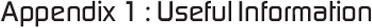Home  -  About the Author  -  About Pukka Press  -  FAQ  -  Contact  -  Buy the BookWhat follows is various and very useful information for reference purposes when constructing and maintaining a grow room.

# Lineal Measurements

Common Use Imperial
· 2 inches = 1 foot
· 3 feet = 1 yard
· 1760 yards = 1 mile

Other Imperial Values
· 12 inches (ins or “) = 1 foot (ft or ‘)
· 3 feet = 1 yard (yd)
· 22 yards = 1 chain (ch)
· 10 chains = 1 furlong (fgs)
· 8 furlongs = 1 mile (mi)

Common Metric Measure
· 10 millimetres (mm) = 1 centimetre (cm)
· 1 metre (mtr) = 1000 millimetres
· 1 metre = 100 centimetres
· 1000 metres = 1 kilometre (km)

Conversions
· 1 inch = 25.4 millimetres
· 1 inch – 2.54 centimetres
· 1 foot = 304.8 millimetres
· 1 foot = 30.48 centimetres
· 1 foot = 0.3048 metres
· 1 yard = 9144 millimetres
· 1 yard = 914.4 centimetres
· 1 yard = 0.9144 metres
· 1 mile = 1609.34 metres
· 1 mile = 1.60934 kilometres
· 1 millimetre = 0.03937 inches
· 1 centimetre = 0.3937 inches
· 1 metre = 39.37 inches
· 1 metre = 3.28084 feet

· 1 metre = 1.0936 yards
· 1 kilometre = 39,370 inches
· 1 kilometre = 3280.84 feet
· 1 kilometre = 1093.613 yards
· 1 kilometre = 0.621317 miles

## Area Measurements

Common Use Imperial
· 144 sq ins (sq”) = 1 sq ft (sq’)
· 9 sq’ = 1 sq yd
· 4840 sq yds = 1 acre
· 640 acres = 1 sq mile

Common Metric Measure
· 10 mm2 = 1 cm2
· 10,000 cm2 = 1 m2
· 1,000,000 m2 = 1 hectare (h)

Conversions
· 1 sq in = 6.45160 cm2
· 1 sq ft = 0.09290 m2
· 1 sq yd = 0.83613 m2
· 1 acre = 0.40469 h
· 1 sq mile = 2.58999 km2
· 1 cm2 = 0.155 sq”
· 1 m2 = 10.76391 sq’
· 1 m2 = 1.19599 sq yds
· 1 h = 2.47105 acres
· 1 km2 = 0.38610 sq miles

## Volume Measurements

Common Use Imperial
· 1728 cu ins = 1 cu ft
· 27 cu ft = 1 cu yd

Common Metric Measure
· 1000 mm3 = 1 cm3
· 1,000,000 cm3 = 1 m3Home  -  About the Author  -  About Pukka Press  -  FAQ  -  Contact  -  Buy the Book

Copyright © 2008 Medical Marijuana Cannabis Cultivation - Pukka Press Ltd. - Disclaimer

All footage taken was filmed on location at the University of London, who possess a Home Office licence to cultivate Cannabis.
All Cannabis grown was grown under this Home Office licence for medical research under a group European initiative to validate the medicinal properties of Cannabis.